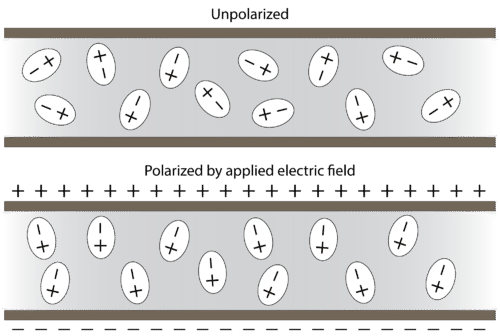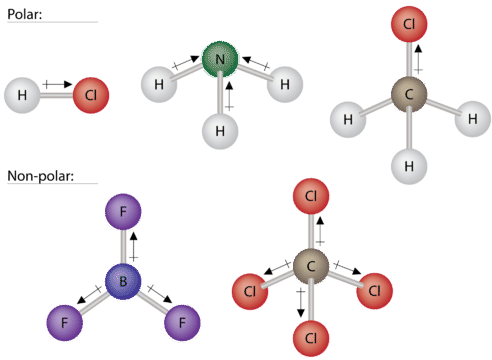# 9.7 Polar Molecules

$$\newcommand{\vecs}{\overset { \rightharpoonup} {\mathbf{#1}} }$$ $$\newcommand{\vecd}{\overset{-\!-\!\rightharpoonup}{\vphantom{a}\smash {#1}}}$$$$\newcommand{\id}{\mathrm{id}}$$ $$\newcommand{\Span}{\mathrm{span}}$$ $$\newcommand{\kernel}{\mathrm{null}\,}$$ $$\newcommand{\range}{\mathrm{range}\,}$$ $$\newcommand{\RealPart}{\mathrm{Re}}$$ $$\newcommand{\ImaginaryPart}{\mathrm{Im}}$$ $$\newcommand{\Argument}{\mathrm{Arg}}$$ $$\newcommand{\norm}{\| #1 \|}$$ $$\newcommand{\inner}{\langle #1, #2 \rangle}$$ $$\newcommand{\Span}{\mathrm{span}}$$ $$\newcommand{\id}{\mathrm{id}}$$ $$\newcommand{\Span}{\mathrm{span}}$$ $$\newcommand{\kernel}{\mathrm{null}\,}$$ $$\newcommand{\range}{\mathrm{range}\,}$$ $$\newcommand{\RealPart}{\mathrm{Re}}$$ $$\newcommand{\ImaginaryPart}{\mathrm{Im}}$$ $$\newcommand{\Argument}{\mathrm{Arg}}$$ $$\newcommand{\norm}{\| #1 \|}$$ $$\newcommand{\inner}{\langle #1, #2 \rangle}$$ $$\newcommand{\Span}{\mathrm{span}}$$Figure $$\PageIndex{1}$$ (Credit: Courtesy of G. Kuebler/JILA and the NIST; Source: http://patapsco.nist.gov/ImageGallery/details.cfm?imageid=583; License: Public Domain)

### How cold are ultracold polar molecules?

Ultracold polar molecules have been created by research scientists. These "molecules" consist of $$\ce{Rb}$$ and $$\ce{K}$$ atoms excited by lasers to form a type of $$\ce{Rb-K}$$ compound where the $$\ce{Rb}$$ has a positive charge and the $$\ce{K}$$ has a negative charge. The material is formed at temperatures extremely close to absolute zero. The researchers believe these techniques will help them make new reactions and new materials.

## Polar Molecules

A polar molecule is a molecule in which one end of the molecule is slightly positive, while the other end is slightly negative. A diatomic molecule that consists of a polar covalent bond, such as $$\ce{HF}$$, is a polar molecule. The two electrically charged regions on either end of the molecule are called poles, similar to a magnet having a north and a south pole. A molecule with two poles is called a dipole (see figure below). Hydrogen fluoride is a dipole.Figure $$\PageIndex{2}$$: A dipole is any molecule with a positive end and a negative end, resulting from unequal distribution of electron density throughout the molecule. (Credit: Christopher Auyeung; Source: CK-12 Foundation; License: CC BY-NC 3.0)

Polar molecules orient themselves in the presence of an electric field with the positive ends of the molecule being attracted to the negative plate, while the negative ends of the molecules are attracted to the positive plate (see figure below).Figure $$\PageIndex{3}$$: Polar molecules are randomly oriented in the absence of an applied electric field (top). In an electric field, the molecules orient themselves because of the attraction of opposite charges (bottom). (Credit: Zachary Wilson; Source: CK-12 Foundation; License: CC BY-NC 3.0)

For molecules with more than two atoms, the molecular geometry must also be taken into account when determining if the molecule is polar or nonpolar. The figure below shows a comparison between carbon dioxide and water. Carbon dioxide $$\left( \ce{CO_2} \right)$$ is a linear molecule. The oxygen atoms are more electronegative than the carbon atom, so there are two individual dipoles pointing outward from the $$\ce{C}$$ atom to each $$\ce{O}$$ atom. However, since the dipoles are of equal strength and are oriented this way, they cancel out, and the overall molecular polarity of $$\ce{CO_2}$$ is zero.

Water is a bent molecule because of the two lone pairs on the central oxygen atom. The individual dipoles point from the $$\ce{H}$$ atoms toward the $$\ce{O}$$ atom. Because of the shape, the dipoles do not cancel each other out, and the water molecule is polar. In the figure below, the net dipole is shown in blue and points upward.Figure $$\PageIndex{4}$$: The molecular geometry of a molecule affects its polarity. (Credit: Joy Sheng; Source: CK-12 Foundation; License: CC BY-NC 3.0)

Some other molecules are shown in the figure below. Notice that a tetrahedral molecule such as $$\ce{CH_4}$$ is nonpolar. However, if one of the peripheral $$\ce{H}$$ atoms is replaced with another atom that has a different electronegativity, the molecule becomes polar. A trigonal planar molecule $$\left( \ce{BF_3} \right)$$ may be nonpolar if all three peripheral atoms are the same, but a trigonal pyramidal molecule $$\left( \ce{NH_3} \right)$$ is polar.Figure $$\PageIndex{5}$$: Some examples of polar and nonpolar molecules based on molecular geometry. (Credit: Joy Sheng; Source: CK-12 Foundation; License: CC BY-NC 3.0)
##### Simulations

Why does water form droplets? How does soap get our clothes clean? Hint: both actions have to do with polarity. Try these simulations to learn more:

How are stains removed from your clothing?

Why do liquids form droplets?

## Summary

• Polar molecules result from differences in the electronegativity of the atoms in the molecule.
• Dipoles that are directly opposite one another cancel each other out.

## Review

1. Why is a polar molecule called a dipole?
2. The molecular geometry of NH3 is trigonal pyramidal. What about the molecular geometry of NH3 makes it a polar molecule?
3. What happens to polar molecules when an electric field is applied?

9.7 Polar Molecules is shared under a CC BY-NC license and was authored, remixed, and/or curated by LibreTexts.